Anzeige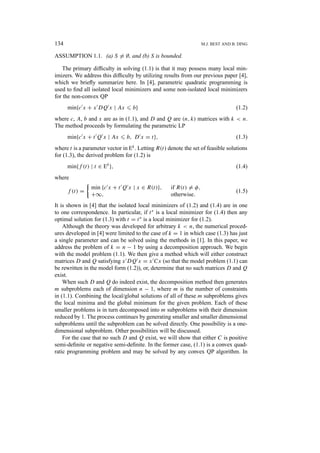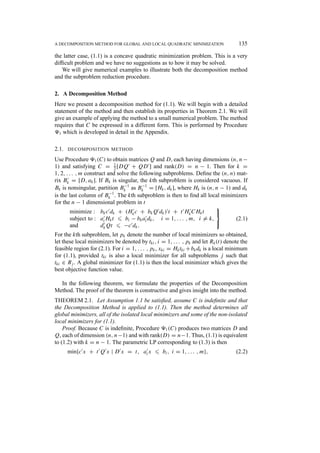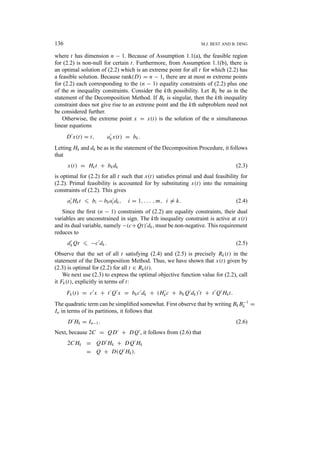Anzeige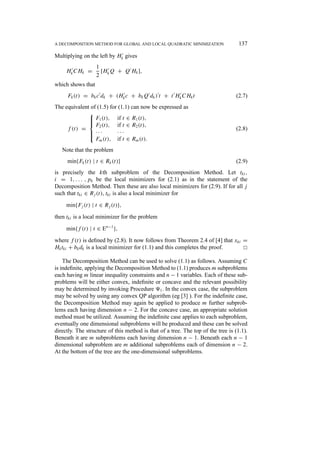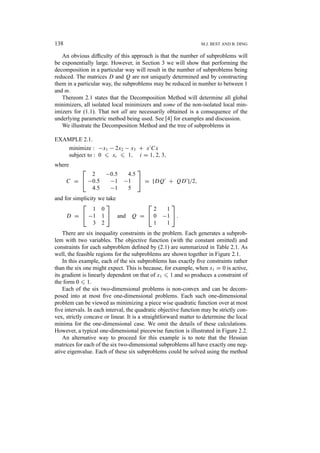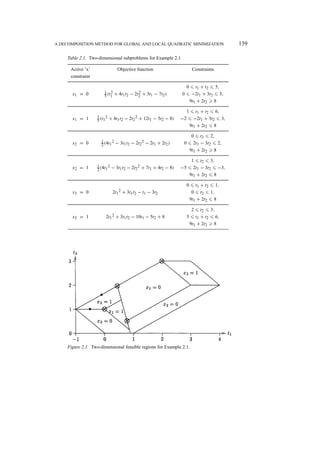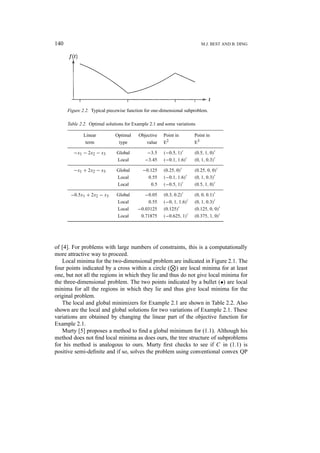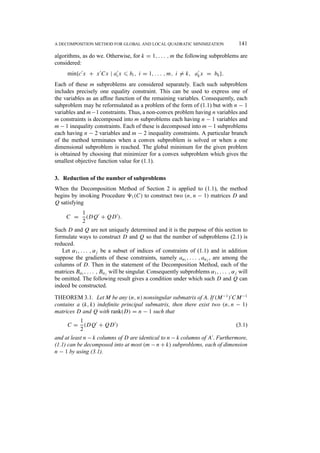Anzeige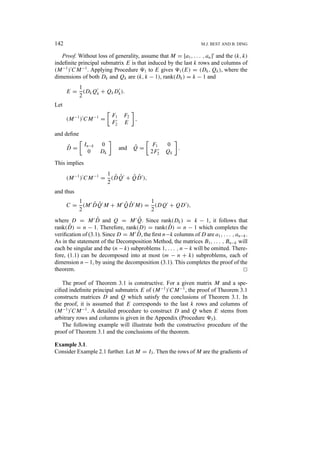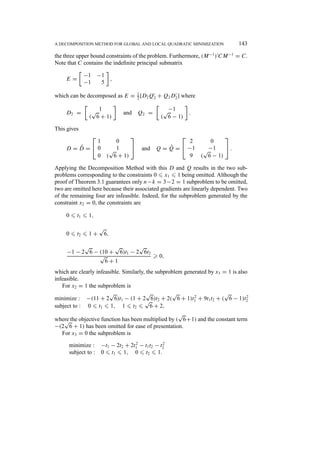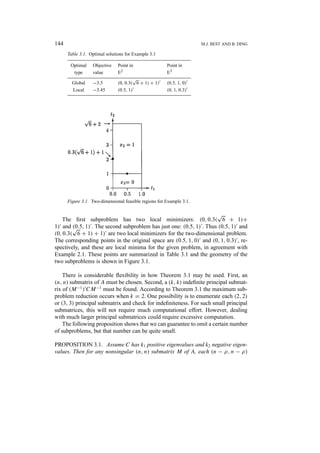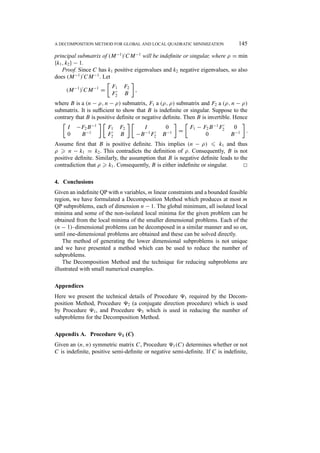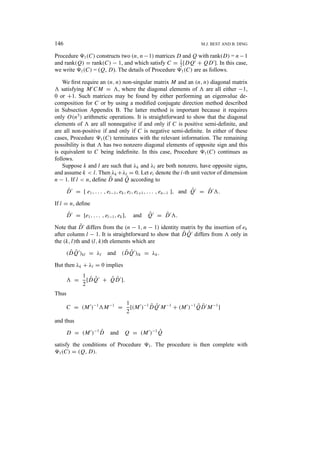Anzeige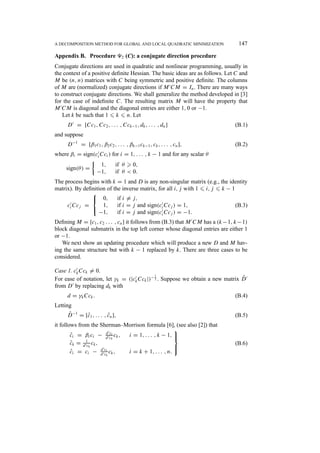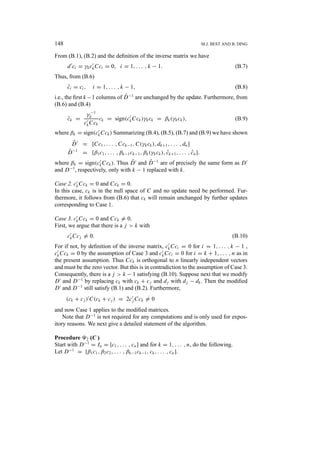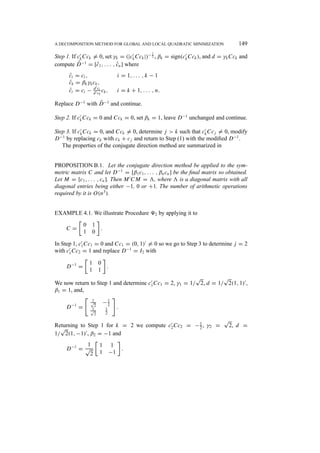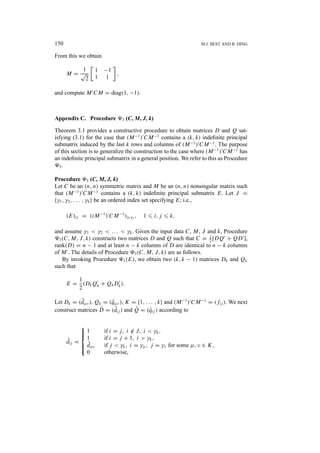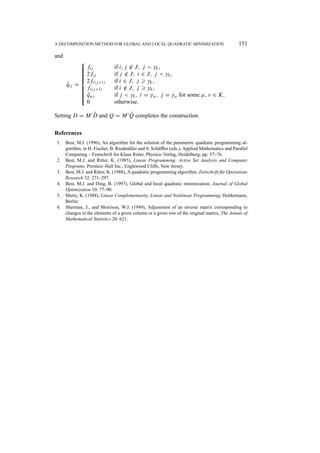AnzeigeNächste SlideShare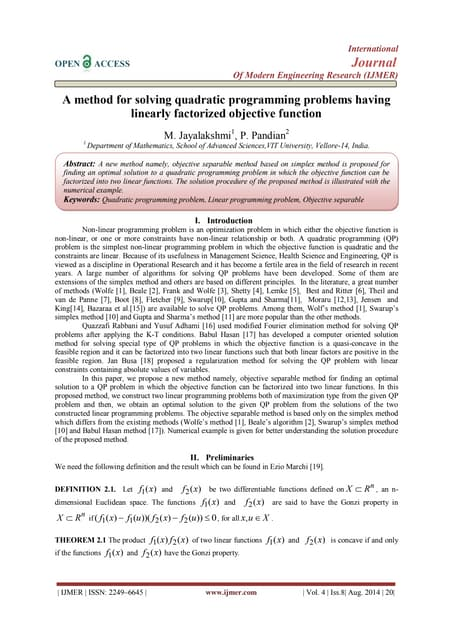A method for solving quadratic programming problems having linearly factoriz...
1 von 20
Anzeige

### a decomposition methodMin quasdratic.pdf

1. Journal of Global Optimization 16: 133–151, 2000. © 2000 Kluwer Academic Publishers. Printed in the Netherlands. 133 A Decomposition Method for Global and Local Quadratic Minimization ? M.J. BEST and B. DING Department of Combinatorics and Optimization, University of Waterloo, Waterloo, Ontario N2L 3G1, Canada (e-mail: mjbest@math.uwaterloo.ca, brian.ding@royalbank.com) (Received 23 August 1996; accepted in final form 22 June 1999) Abstract. We present a decomposition method for indefinite quadratic programming problems having n variables and m linear constraints. The given problem is decomposed into at most m QP subproblems each having m linear constraints and n − 1 variables. All global minima, all isolated local minima and some of the non-isolated local minima for the given problem are obtained from those of the lower dimensional subproblems. One way to continue solving the given problem is to apply the decomposition method again to the subproblems and repeatedly doing so until subproblems of dimension 1 are produced and these can be solved directly. A technique to reduce the potentially large number of subproblems is formulated. Key words: Local and global optimization, Non-convex quadratic program, Parametric linear pro- gramming. 1. Introduction We propose a method to solve min{c0 x + x0 Cx | Ax 6 b}, (1.1) where C is (n, n), symmetric and indefinite, c and x are n-vectors, A is an (m, n) matrix and b is an m-vector. Matrix transposition is denoted by a prime (0 ) and all non-primed vectors will be assumed to be column vectors. In will denote the (n, n) identity matrix and En will denote Euclidean n-space. Let S = {x | Ax 6 b} denote the feasible region for (1.1). When it is necessary to discuss the individual constraints for (1.1), we will write S in the form S = {x | a0 ix 6 bi, i = 1, . . . , m}, so that a0 i denotes the ith row of A. We require the following to be satisfied throughout this paper. ? This research was supported by the National Sciences and Engineering Research Council of Canada under Grant A8189.
2. 134 M.J. BEST AND B. DING ASSUMPTION 1.1. (a) S 6= ∅, and (b) S is bounded. The primary difficulty in solving (1.1) is that it may possess many local min- imizers. We address this difficulty by utilizing results from our previous paper , which we briefly summarize here. In , parametric quadratic programming is used to find all isolated local minimizers and some non-isolated local minimizers for the non-convex QP min{c0 x + x0 DQ0 x | Ax 6 b} (1.2) where c, A, b and x are as in (1.1), and D and Q are (n, k) matrices with k < n. The method proceeds by formulating the parametric LP min{c0 x + t0 Q0 x | Ax 6 b, D0 x = t}, (1.3) where t is a parameter vector in Ek . Letting R(t) denote the set of feasible solutions for (1.3), the derived problem for (1.2) is min{f (t) | t ∈ Ek }, (1.4) where f (t) = min {c0 x + t0 Q0 x | x ∈ R(t)}, if R(t) 6= φ, +∞, otherwise. (1.5) It is shown in  that the isolated local minimizers of (1.2) and (1.4) are in one to one correspondence. In particular, if t∗ is a local minimizer for (1.4) then any optimal solution for (1.3) with t = t∗ is a local minimizer for (1.2). Although the theory was developed for arbitrary k n, the numerical proced- ures developed in  were limited to the case of k = 1 in which case (1.3) has just a single parameter and can be solved using the methods in . In this paper, we address the problem of k = n − 1 by using a decomposition approach. We begin with the model problem (1.1). We then give a method which will either construct matrices D and Q satisfying x0 DQ0 x = x0 Cx (so that the model problem (1.1) can be rewritten in the model form (1.2)), or, determine that no such matrices D and Q exist. When such D and Q do indeed exist, the decomposition method then generates m subproblems each of dimension n − 1, where m is the number of constraints in (1.1). Combining the local/global solutions of all of these m subproblems gives the local minima and the global minimum for the given problem. Each of these smaller problems is in turn decomposed into m subproblems with their dimension reduced by 1. The process continues by generating smaller and smaller dimensional subproblems until the subproblem can be solved directly. One possibility is a one- dimensional subproblem. Other possibilities will be discussed. For the case that no such D and Q exist, we will show that either C is positive semi-definite or negative semi-definite. In the former case, (1.1) is a convex quad- ratic programming problem and may be solved by any convex QP algorithm. In
3. A DECOMPOSITION METHOD FOR GLOBAL AND LOCAL QUADRATIC MINIMIZATION 135 the latter case, (1.1) is a concave quadratic minimization problem. This is a very difficult problem and we have no suggestions as to how it may be solved. We will give numerical examples to illustrate both the decomposition method and the subproblem reduction procedure. 2. A Decomposition Method Here we present a decomposition method for (1.1). We will begin with a detailed statement of the method and then establish its properties in Theorem 2.1. We will give an example of applying the method to a small numerical problem. The method requires that C be expressed in a different form. This is performed by Procedure 91 which is developed in detail in the Appendix. 2.1. DECOMPOSITION METHOD Use Procedure 91(C) to obtain matrices Q and D, each having dimensions (n, n− 1) and satisfying C = 1 2 [DQ0 + QD0 ] and rank(D) = n − 1. Then for k = 1, 2, . . . , m construct and solve the following subproblems. Define the (n, n) mat- rix B0 k = [D, ak]. If Bk is singular, the kth subproblem is considered vacuous. If Bk is nonsingular, partition B−1 k as B−1 k = [Hk, dk], where Hk is (n, n − 1) and dk is the last column of B−1 k . The kth subproblem is then to find all local minimizers for the n − 1 dimensional problem in t minimize : bkc0 dk + (H0 kc + bkQ0 dk)0 t + t0 H0 kCHkt subject to : a0 iHkt 6 bi − bka0 idk, i = 1, . . . , m, i 6= k, and d0 kQt 6 −c0 dk.    (2.1) For the kth subproblem, let pk denote the number of local minimizers so obtained, let these local minimizers be denoted by tki, i = 1, . . . , pk and let Rk(t) denote the feasible region for (2.1). For i = 1, . . . , pk, xki = Hktki +bkdk is a local minimum for (1.1), provided tki is also a local minimizer for all subproblems j such that tki ∈ Rj . A global minimizer for (1.1) is then the local minimizer which gives the best objective function value. In the following theorem, we formulate the properties of the Decomposition Method. The proof of the theorem is constructive and gives insight into the method. THEOREM 2.1. Let Assumption 1.1 be satisfied, assume C is indefinite and that the Decomposition Method is applied to (1.1). Then the method determines all global minimizers, all of the isolated local minimizers and some of the non-isolated local minimizers for (1.1). Proof. Because C is indefinite, Procedure 91(C) produces two matrices D and Q, each of dimension (n, n−1) and with rank(D) = n−1. Thus, (1.1) is equivalent to (1.2) with k = n − 1. The parametric LP corresponding to (1.3) is then min{c0 x + t0 Q0 x | D0 x = t, a0 ix 6 bi, i = 1, . . . , m}, (2.2)
4. 136 M.J. BEST AND B. DING where t has dimension n − 1. Because of Assumption 1.1(a), the feasible region for (2.2) is non-null for certain t. Furthermore, from Assumption 1.1(b), there is an optimal solution of (2.2) which is an extreme point for all t for which (2.2) has a feasible solution. Because rank(D) = n − 1, there are at most m extreme points for (2.2) each corresponding to the (n − 1) equality constraints of (2.2) plus one of the m inequality constraints. Consider the kth possibility. Let Bk be as in the statement of the Decomposition Method. If Bk is singular, then the kth inequality constraint does not give rise to an extreme point and the kth subproblem need not be considered further. Otherwise, the extreme point x = x(t) is the solution of the n simultaneous linear equations D0 x(t) = t, a0 kx(t) = bk. Letting Hk and dk be as in the statement of the Decomposition Procedure, it follows that x(t) = Hkt + bkdk (2.3) is optimal for (2.2) for all t such that x(t) satisfies primal and dual feasibility for (2.2). Primal feasibility is accounted for by substituting x(t) into the remaining constraints of (2.2). This gives a0 iHkt 6 bi − bka0 idk, i = 1, . . . , m, i 6= k. (2.4) Since the first (n − 1) constraints of (2.2) are equality constraints, their dual variables are unconstrained in sign. The kth inequality constraint is active at x(t) and its dual variable, namely −(c+Qt)0 dk, must be non-negative. This requirement reduces to d0 kQt 6 −c0 dk. (2.5) Observe that the set of all t satisfying (2.4) and (2.5) is precisely Rk(t) in the statement of the Decomposition Method. Thus, we have shown that x(t) given by (2.3) is optimal for (2.2) for all t ∈ Rk(t). We next use (2.3) to express the optimal objective function value for (2.2), call it Fk(t), explicitly in terms of t: Fk(t) = c0 x + t0 Q0 x = bkc0 dk + (H0 kc + bkQ0 dk)0 t + t0 Q0 Hkt. The quadratic term can be simplified somewhat. First observe that by writing BkB−1 k = In in terms of its partitions, it follows that D0 Hk = In−1. (2.6) Next, because 2C = QD0 + DQ0 , it follows from (2.6) that 2CHk = QD0 Hk + DQ0 Hk = Q + D(Q0 Hk).
5. A DECOMPOSITION METHOD FOR GLOBAL AND LOCAL QUADRATIC MINIMIZATION 137 Multiplying on the left by H0 k gives H0 kCHk = 1 2 [H0 kQ + Q0 Hk], which shows that Fk(t) = bkc0 dk + (H0 kc + bkQ0 dk)0 t + t0 H0 kCHkt (2.7) The equivalent of (1.5) for (1.1) can now be expressed as f (t) =        F1(t), if t ∈ R1(t), F2(t), if t ∈ R2(t), · · · · · · Fm(t), if t ∈ Rm(t). (2.8) Note that the problem min{Fk(t) | t ∈ Rk(t)} (2.9) is precisely the kth subproblem of the Decomposition Method. Let tki, i = 1, . . . , pk be the local minimizers for (2.1) as in the statement of the Decomposition Method. Then these are also local minimizers for (2.9). If for all j such that tki ∈ Rj (t), tki is also a local minimizer for min{Fj (t) | t ∈ Rj (t)}, then tki is a local minimizer for the problem min{f (t) | t ∈ En−1 }, where f (t) is defined by (2.8). It now follows from Theorem 2.4 of  that xki = Hktki + bkdk is a local minimizer for (1.1) and this completes the proof. 2 The Decomposition Method can be used to solve (1.1) as follows. Assuming C is indefinite, applying the Decomposition Method to (1.1) produces m subproblems each having m linear inequality constraints and n − 1 variables. Each of these sub- problems will be either convex, indefinite or concave and the relevant possibility may be determined by invoking Procedure 91. In the convex case, the subproblem may be solved by using any convex QP algorithm (eg  ). For the indefinite case, the Decomposition Method may again be applied to produce m further subprob- lems each having dimension n − 2. For the concave case, an appropriate solution method must be utilized. Assuming the indefinite case applies to each subproblem, eventually one dimensional subproblems will be produced and these can be solved directly. The structure of this method is that of a tree. The top of the tree is (1.1). Beneath it are m subproblems each having dimension n − 1. Beneath each n − 1 dimensional subproblem are m additional subproblems each of dimension n − 2. At the bottom of the tree are the one-dimensional subproblems.
6. 138 M.J. BEST AND B. DING An obvious difficulty of this approach is that the number of subproblems will be exponentially large. However, in Section 3 we will show that performing the decomposition in a particular way will result in the number of subproblems being reduced. The matrices D and Q are not uniquely determined and by constructing them in a particular way, the subproblems may be reduced in number to between 1 and m. Thereom 2.1 states that the Decomposition Method will determine all global minimizers, all isolated local minimizers and some of the non-isolated local min- imizers for (1.1). That not all are necessarily obtained is a consequence of the underlying parametric method being used. See  for examples and discussion. We illustrate the Decomposition Method and the tree of subproblems in EXAMPLE 2.1. minimize : −x1 − 2x2 − x3 + x0 Cx subject to : 0 6 xi 6 1, i = 1, 2, 3, where C =   2 −0.5 4.5 −0.5 −1 −1 4.5 −1 5   = [DQ0 + QD0 ]/2, and for simplicity we take D =   1 0 −1 1 3 2   and Q =   2 1 0 −1 1 1   . There are six inequality constraints in the problem. Each generates a subprob- lem with two variables. The objective function (with the constant omitted) and constraints for each subproblem defined by (2.1) are summarized in Table 2.1. As well, the feasible regions for the subproblems are shown together in Figure 2.1. In this example, each of the six subproblems has exactly five constraints rather than the six one might expect. This is because, for example, when x1 = 0 is active, its gradient is linearly dependent on that of x1 6 1 and so produces a constraint of the form 0 6 1. Each of the six two-dimensional problems is non-convex and can be decom- posed into at most five one-dimensional problems. Each such one-dimensional problem can be viewed as minimizing a piece wise quadratic function over at most five intervals. In each interval, the quadratic objective function may be strictly con- vex, strictly concave or linear. It is a straightforward matter to determine the local minima for the one-dimensional case. We omit the details of these calculations. However, a typical one-dimensional piecewise function is illustrated in Figure 2.2. An alternative way to proceed for this example is to note that the Hessian matrices for each of the six two-dimensional subproblems all have exactly one neg- ative eigenvalue. Each of these six subproblems could be solved using the method
7. A DECOMPOSITION METHOD FOR GLOBAL AND LOCAL QUADRATIC MINIMIZATION 139 Table 2.1. Two-dimensional subproblems for Example 2.1 Active ’x’ Objective function Constraints constraint 0 6 t1 + t2 6 5, x1 = 0 1 5 (t2 1 + 4t1t2 − 2t2 2 + 3t1 − 7t2) 0 6 −2t1 + 3t2 6 5, 9t1 + 2t2 8 1 6 t1 + t2 6 6, x1 = 1 1 5 (t1 2 + 4t1t2 − 2t2 2 + 12t1 − 5t2 − 8) −2 6 −2t1 + 3t2 6 3, 9t1 + 2t2 6 8 0 6 t2 6 2, x2 = 0 1 2(4t1 2 − 3t1t2 − 2t2 2 − 2t1 + 2t2) 0 6 2t1 − 3t2 6 2, 9t1 + 2t2 8 1 6 t2 6 3, x2 = 1 1 2 (4t1 2 − 3t1t2 − 2t2 2 + 7t1 + 4t2 − 8) −5 6 2t1 − 3t2 6 −3, 9t1 + 2t2 6 8 0 6 t1 + t2 6 1, x3 = 0 2t1 2 + 3t1t2 − t1 − 3t2 0 6 t2 6 1, 9t1 + 2t2 6 8 2 6 t2 6 3, x3 = 1 2t1 2 + 3t1t2 − 10t1 − 5t2 + 8 5 6 t1 + t2 6 6, 9t1 + 2t2 8 Figure 2.1. Two-dimensional feasible regions for Example 2.1.
8. 140 M.J. BEST AND B. DING Figure 2.2. Typical piecewise function for one-dimensional subproblem. Table 2.2. Optimal solutions for Example 2.1 and some variations Linear Optimal Objective Point in Point in term type value E2 E3 −x1 − 2x2 − x3 Global −3.5 (−0.5, 1)0 (0.5, 1, 0)0 Local −3.45 (−0.1, 1.6)0 (0, 1, 0.3)0 −x1 + 2x2 − x3 Global −0.125 (0.25, 0)0 (0.25, 0, 0)0 Local 0.55 (−0.1, 1.6)0 (0, 1, 0.3)0 Local 0.5 (−0.5, 1)0 (0.5, 1, 0)0 −0.5x1 + 2x2 − x3 Global −0.05 (0.3, 0.2)0 (0, 0, 0.1)0 Local 0.55 (−0, 1, 1.6)0 (0, 1, 0.3)0 Local −0.03125 (0.125)0 (0.125, 0, 0)0 Local 0.71875 (−0.625, 1)0 (0.375, 1, 0)0 of . For problems with large numbers of constraints, this is a computationally more attractive way to proceed. Local minima for the two-dimensional problem are indicated in Figure 2.1. The four points indicated by a cross within a circle ( N ) are local minima for at least one, but not all the regions in which they lie and thus do not give local minima for the three-dimensional problem. The two points indicated by a bullet (•) are local minima for all the regions in which they lie and thus give local minima for the original problem. The local and global minimizers for Example 2.1 are shown in Table 2.2. Also shown are the local and global solutions for two variations of Example 2.1. These variations are obtained by changing the linear part of the objective function for Example 2.1. Murty  proposes a method to find a global minimum for (1.1). Although his method does not find local minima as does ours, the tree structure of subproblems for his method is analogous to ours. Murty first checks to see if C in (1.1) is positive semi-definite and if so, solves the problem using conventional convex QP
9. A DECOMPOSITION METHOD FOR GLOBAL AND LOCAL QUADRATIC MINIMIZATION 141 algorithms, as do we. Otherwise, for k = 1, . . . , m the following subproblems are considered: min{c0 x + x0 Cx | a0 ix 6 bi, i = 1, . . . , m, i 6= k, a0 kx = bk}. Each of these m subproblems are considered separately. Each such subproblem includes precisely one equality constraint. This can be used to express one of the variables as an affine function of the remaining variables. Consequently, each subproblem may be reformulated as a problem of the form of (1.1) but with n − 1 variables and m−1 constraints. Thus, a non-convex problem having n variables and m constraints is decomposed into m subproblems each having n − 1 variables and m − 1 inequality constraints. Each of these is decomposed into m − 1 subproblems each having n − 2 variables and m − 2 inequality constraints. A particular branch of the method terminates when a convex subproblem is solved or when a one dimensional subproblem is reached. The global minimum for the given problem is obtained by choosing that minimizer for a convex subproblem which gives the smallest objective function value for (1.1). 3. Reduction of the number of subproblems When the Decomposition Method of Section 2 is applied to (1.1), the method begins by invoking Procedure 91(C) to construct two (n, n − 1) matrices D and Q satisfying C = 1 2 (DQ0 + QD0 ). Such D and Q are not uniquely determined and it is the purpose of this section to formulate ways to construct D and Q so that the number of subproblems (2.1) is reduced. Let α1, . . . , αj be a subset of indices of constraints of (1.1) and in addition suppose the gradients of these constraints, namely aα1 , . . . , aαj , are among the columns of D. Then in the statement of the Decomposition Method, each of the matrices Bα1 , . . . , Bαj will be singular. Consequently subproblems α1, . . . , αj will be omitted. The following result gives a condition under which such D and Q can indeed be constructed. THEOREM 3.1. Let M be any (n, n) nonsingular submatrix of A. If (M−1 )0 CM−1 contains a (k, k) indefinite principal submatrix, then there exist two (n, n − 1) matrices D and Q with rank(D) = n − 1 such that C = 1 2 (DQ0 + QD0 ) (3.1) and at least n − k columns of D are identical to n − k columns of A0 . Furthermore, (1.1) can be decomposed into at most (m − n + k) subproblems, each of dimension n − 1 by using (3.1).
10. 142 M.J. BEST AND B. DING Proof. Without loss of generality, assume that M = [a1, . . . , an]0 and the (k, k) indefinite principal submatrix E is that induced by the last k rows and columns of (M−1 )0 CM−1 . Applying Procedure 91 to E gives 91(E) = (Dk, Qk), where the dimensions of both Dk and Qk are (k, k − 1), rank(Dk) = k − 1 and E = 1 2 (DkQ0 k + QkD0 k). Let (M−1 )0 CM−1 = F1 F2 F0 2 E , and define D̂ = In−k 0 0 Dk and Q̂ = F1 0 2F0 2 Qk . This implies (M−1 )0 CM−1 = 1 2 (D̂Q̂0 + Q̂D̂0 ), and thus C = 1 2 (M0 D̂Q̂0 M + M0 Q̂D̂0 M) = 1 2 (DQ0 + QD0 ), where D = M0 D̂ and Q = M0 Q̂. Since rank(Dk) = k − 1, it follows that rank(D̂) = n − 1. Therefore, rank(D) = rank(D̂) = n − 1 which completes the verification of (3.1). Since D = M0 D̂, the first n−k columns of D are a1, . . . , an−k. As in the statement of the Decomposition Method, the matrices B1, . . . , Bn−k will each be singular and the (n − k) subproblems 1, . . . , n − k will be omitted. There- fore, (1.1) can be decomposed into at most (m − n + k) subproblems, each of dimension n−1, by using the decomposition (3.1). This completes the proof of the theorem. 2 The proof of Theorem 3.1 is constructive. For a given matrix M and a spe- cified indefinite principal submatrix E of (M−1 )0 CM−1 , the proof of Theorem 3.1 constructs matrices D and Q which satisfy the conclusions of Theorem 3.1. In the proof, it is assumed that E corresponds to the last k rows and columns of (M−1 )0 CM−1 . A detailed procedure to construct D and Q when E stems from arbitrary rows and columns is given in the Appendix (Procedure 93). The following example will illustrate both the constructive procedure of the proof of Theorem 3.1 and the conclusions of the theorem. Example 3.1. Consider Example 2.1 further. Let M = I3. Then the rows of M are the gradients of
11. A DECOMPOSITION METHOD FOR GLOBAL AND LOCAL QUADRATIC MINIMIZATION 143 the three upper bound constraints of the problem. Furthermore, (M−1 )0 CM−1 = C. Note that C contains the indefinite principal submatrix E = −1 −1 −1 5 , which can be decomposed as E = 1 2 [D2Q0 2 + Q2D0 2] where D2 = 1 ( √ 6 + 1) and Q2 = −1 ( √ 6 − 1) . This gives D = D̂ =   1 0 0 1 0 ( √ 6 + 1)   and Q = Q̂ =   2 0 −1 −1 9 ( √ 6 − 1)   . Applying the Decomposition Method with this D and Q results in the two sub- problems corresponding to the constraints 0 6 x1 6 1 being omitted. Although the proof of Theorem 3.1 guarantees only n−k = 3−2 = 1 subproblem to be omitted, two are omitted here because their associated gradients are linearly dependent. Two of the remaining four are infeasible. Indeed, for the subproblem generated by the constraint x2 = 0, the constraints are 0 6 t1 6 1, 0 6 t2 6 1 + √ 6, −1 − 2 √ 6 − (10 + √ 6)t1 − 2 √ 6t2 √ 6 + 1 0, which are clearly infeasible. Similarly, the subproblem generated by x3 = 1 is also infeasible. For x2 = 1 the subproblem is minimize : −(11 + 2 √ 6)t1 − (1 + 2 √ 6)t2 + 2( √ 6 + 1)t2 1 + 9t1t2 + ( √ 6 − 1)t2 2 subject to : 0 6 t1 6 1, 1 6 t2 6 √ 6 + 2, where the objective function has been multiplied by ( √ 6+1) and the constant term −(2 √ 6 + 1) has been omitted for ease of presentation. For x3 = 0 the subproblem is minimize : −t1 − 2t2 + 2t2 1 − t1t2 − t2 2 subject to : 0 6 t1 6 1, 0 6 t2 6 1.
12. 144 M.J. BEST AND B. DING Table 3.1. Optimal solutions for Example 3.1 Optimal Objective Point in Point in type value E2 E3 Global −3.5 (0, 0.3( √ 6 + 1) + 1)0 (0.5, 1, 0)0 Local −3.45 (0.5, 1)0 (0, 1, 0.3)0 Figure 3.1. Two-dimensional feasible regions for Example 3.1. The first subproblem has two local minimizers: (0, 0.3( √ 6 + 1)+ 1)0 and (0.5, 1)0 . The second subproblem has just one: (0.5, 1)0 . Thus (0.5, 1)0 and (0, 0.3( √ 6 + 1) + 1)0 are two local minimizers for the two-dimensional problem. The corresponding points in the original space are (0.5, 1, 0)0 and (0, 1, 0.3)0 , re- spectively, and these are local minima for the given problem, in agreement with Example 2.1. These points are summarized in Table 3.1 and the geometry of the two subproblems is shown in Figure 3.1. There is considerable flexibility in how Theorem 3.1 may be used. First, an (n, n) submatrix of A must be chosen. Second, a (k, k) indefinite principal submat- rix of (M−1 )0 CM−1 must be found. According to Theorem 3.1 the maximum sub- problem reduction occurs when k = 2. One possibility is to enumerate each (2, 2) or (3, 3) principal submatrix and check for indefiniteness. For such small principal submatrices, this will not require much computational effort. However, dealing with much larger principal submatrices could require excessive computation. The following proposition shows that we can guarantee to omit a certain number of subproblems, but that number can be quite small. PROPOSITION 3.1. Assume C has k1 positive eigenvalues and k2 negative eigen- values. Then for any nonsingular (n, n) submatrix M of A, each (n − ρ, n − ρ)
13. A DECOMPOSITION METHOD FOR GLOBAL AND LOCAL QUADRATIC MINIMIZATION 145 principal submatrix of (M−1 )0 CM−1 will be indefinite or singular, where ρ = min {k1, k2} − 1. Proof. Since C has k1 positive eigenvalues and k2 negative eigenvalues, so also does (M−1 )0 CM−1 . Let (M−1 )0 CM−1 = F1 F2 F0 2 B , where B is a (n − ρ, n − ρ) submatrix, F1 a (ρ, ρ) submatrix and F2 a (ρ, n − ρ) submatrix. It is sufficient to show that B is indefinite or singular. Suppose to the contrary that B is positive definite or negative definite. Then B is invertible. Hence I −F2B−1 0 B−1 F1 F2 F0 2 B I 0 −B−1 F0 2 B−1 = F1 − F2B−1 F0 2 0 0 B−1 . Assume first that B is positive definite. This implies (n − ρ) 6 k1 and thus ρ n − k1 = k2. This contradicts the definition of ρ. Consequently, B is not positive definite. Similarly, the assumption that B is negative definite leads to the contradiction that ρ k1. Consequently, B is either indefinite or singular. 2 4. Conclusions Given an indefinite QP with n variables, m linear constraints and a bounded feasible region, we have formulated a Decomposition Method which produces at most m QP subproblems, each of dimension n − 1. The global minimum, all isolated local minima and some of the non-isolated local minima for the given problem can be obtained from the local minima of the smaller dimensional problems. Each of the (n − 1)–dimensional problems can be decomposed in a similar manner and so on, until one-dimensional problems are obtained and these can be solved directly. The method of generating the lower dimensional subproblems is not unique and we have presented a method which can be used to reduce the number of subproblems. The Decomposition Method and the technique for reducing subproblems are illustrated with small numerical examples. Appendices Here we present the technical details of Procedure 91 required by the Decom- position Method, Procedure 92 (a conjugate direction procedure) which is used by Procedure 91, and Procedure 93 which is used in reducing the number of subproblems for the Decomposition Method. Appendix A. Procedure 91 (C) Given an (n, n) symmetric matrix C, Procedure 91(C) determines whether or not C is indefinite, positive semi-definite or negative semi-definite. If C is indefinite,
14. 146 M.J. BEST AND B. DING Procedure 91(C) constructs two (n, n−1) matrices D and Q with rank(D) = n−1 and rank(Q) = rank(C) − 1, and which satisfy C = 1 2 [DQ0 + QD0 ]. In this case, we write 91(C) = (Q, D). The details of Procedure 91(C) are as follows. We first require an (n, n) non-singular matrix M and an (n, n) diagonal matrix 3 satisfying M0 CM = 3, where the diagonal elements of 3 are all either −1, 0 or +1. Such matrices may be found by either performing an eigenvalue de- composition for C or by using a modified conjugate direction method described in Subsection Appendix B. The latter method is important because it requires only O(n3 ) arithmetic operations. It is straightforward to show that the diagonal elements of 3 are all nonnegative if and only if C is positive semi-definite, and are all non-positive if and only if C is negative semi-definite. In either of these cases, Procedure 91(C) terminates with the relevant information. The remaining possibility is that 3 has two nonzero diagonal elements of opposite sign and this is equivalent to C being indefinite. In this case, Procedure 91(C) continues as follows. Suppose k and l are such that λk and λl are both nonzero, have opposite signs, and assume k l. Then λk +λl = 0. Let ei denote the i-th unit vector of dimension n − 1. If l n, define D̂ and Q̂ according to D̂0 = [ e1, . . . , el−1, ek, el, el+1, . . . , en−1 ], and Q̂0 = D̂0 3. If l = n, define D̂0 = [e1, . . . , el−1, ek], and Q̂0 = D̂0 3. Note that D̂0 differs from the (n − 1, n − 1) identity matrix by the insertion of ek after column l − 1. It is straightforward to show that D̂Q̂0 differs from 3 only in the (k, l)th and (l, k)th elements which are (D̂Q̂0 )kl = λl and (D̂Q̂0 )lk = λk. But then λk + λl = 0 implies 3 = 1 2 [D̂Q̂0 + Q̂D̂0 ]. Thus C = (M0 )−1 3M−1 = 1 2 [(M0 )−1 D̂Q̂0 M−1 + (M0 )−1 Q̂D̂0 M−1 ] and thus D = (M0 )−1 D̂ and Q = (M0 )−1 Q̂ satisfy the conditions of Procedure 91. The procedure is then complete with 91(C) = (Q, D).
15. A DECOMPOSITION METHOD FOR GLOBAL AND LOCAL QUADRATIC MINIMIZATION 147 Appendix B. Procedure 92 (C): a conjugate direction procedure Conjugate directions are used in quadratic and nonlinear programming, usually in the context of a positive definite Hessian. The basic ideas are as follows. Let C and M be (n, n) matrices with C being symmetric and positive definite. The columns of M are (normalized) conjugate directions if M0 CM = In. There are many ways to construct conjugate directions. We shall generalize the method developed in  for the case of indefinite C. The resulting matrix M will have the property that M0 CM is diagonal and the diagonal entries are either 1, 0 or −1. Let k be such that 1 6 k 6 n. Let D0 = [Cc1, Cc2, . . . , Cck−1, dk, . . . , dn] (B.1) and suppose D−1 = [β1c1, β2c2, . . . , βk−1ck−1, ck, . . . , cn], (B.2) where βi = sign(c0 iCci) for i = 1, . . . , k − 1 and for any scalar θ sign(θ) = 1, if θ 0, −1, if θ 0. The process begins with k = 1 and D is any non-singular matrix (e.g., the identity matrix). By definition of the inverse matrix, for all i, j with 1 6 i, j 6 k − 1 c0 iCcj =    0, if i 6= j, 1, if i = j and sign(c0 iCcj ) = 1, −1, if i = j and sign(c0 iCcj ) = −1. (B.3) Defining M = [c1, c2 . . . , cn] it follows from (B.3) that M0 CM has a (k−1, k−1) block diagonal submatrix in the top left corner whose diagonal entries are either 1 or −1. We next show an updating procedure which will produce a new D and M hav- ing the same structure but with k − 1 replaced by k. There are three cases to be considered. Case 1. c0 kCck 6= 0. For ease of notation, let γk = (|c0 kCck|)− 1 2 . Suppose we obtain a new matrix D̂0 from D0 by replacing dk with d = γkCck. (B.4) Letting D̂−1 = [ĉ1, . . . , ĉn], (B.5) it follows from the Sherman–Morrison formula , (see also ) that ĉi = βici − d0ci d0ck ck, i = 1, . . . , k − 1, ĉk = 1 d0ck ck, ĉi = ci − d0ci d0ck ck, i = k + 1, . . . , n.      (B.6)
16. 148 M.J. BEST AND B. DING From (B.1), (B.2) and the definition of the inverse matrix we have d0 ci = γkc0 kCci = 0, i = 1, . . . , k − 1. (B.7) Thus, from (B.6) ĉi = ci, i = 1, . . . , k − 1, (B.8) i.e., the first k−1 columns of D̂−1 are unchanged by the update. Furthermore, from (B.6) and (B.4) ĉk = γ −1 k c0 kCck ck = sign(c0 kCck)γkck = βk(γkck), (B.9) where βk = sign(c0 kCck) Summarizing (B.4), (B.5), (B.7) and (B.9) we have shown D̂0 = [Cc1, . . . , Cck−1, C(γkck), dk+1, . . . , dn] D̂−1 = [β1c1, . . . , βk−1ck−1, βk(γkck), ĉk+1, . . . , ĉn]. where βk = sign(c0 kCck). Thus D̂0 and D̂−1 are of precisely the same form as D0 and D−1 , respectively, only with k − 1 replaced with k. Case 2. c0 kCck = 0 and Cck = 0. In this case, ck is in the null space of C and no update need be performed. Fur- thermore, it follows from (B.6) that ck will remain unchanged by further updates corresponding to Case 1. Case 3. c0 kCck = 0 and Cck 6= 0. First, we argue that there is a j k with c0 kCcj 6= 0. (B.10) For if not, by definition of the inverse matrix, c0 kCci = 0 for i = 1, . . . , k − 1 , c0 kCck = 0 by the assumption of Case 3 and c0 kCci = 0 for i = k + 1, . . . , n as in the present assumption. Thus Cck is orthogonal to n linearly independent vectors and must be the zero vector. But this is in contradiction to the assumption of Case 3. Consequently, there is a j k − 1 satisfying (B.10). Suppose next that we modify D0 and D−1 by replacing ck with ck + cj and dj with dj − dk. Then the modified D0 and D−1 still satisfy (B.1) and (B.2). Furthermore, (ck + cj )0 C(ck + cj ) = 2c0 j Cck 6= 0 and now Case 1 applies to the modified matrices. Note that D−1 is not required for any computations and is only used for expos- itory reasons. We next give a detailed statement of the algorithm. Procedure 92 (C ) Start with D−1 = In = [c1, . . . , cn] and for k = 1, . . . , n, do the following. Let D−1 = [β1c1, β2c2, . . . , βk−1ck−1, ck, . . . , cn].
17. A DECOMPOSITION METHOD FOR GLOBAL AND LOCAL QUADRATIC MINIMIZATION 149 Step 1. If c0 kCck 6= 0, set γk = (|c0 kCck|)− 1 2 , βk = sign(c0 kCck), and d = γkCck and compute D̂−1 = [ĉ1, . . . , ĉn] where ĉi = ci, i = 1, . . . , k − 1 ĉk = βkγkck, ĉi = ci − d0ci d0ck ck, i = k + 1, . . . , n. Replace D−1 with D̂−1 and continue. Step 2. If c0 kCck = 0 and Cck = 0, set βk = 1, leave D−1 unchanged and continue. Step 3. If c0 kCck = 0, and Cck 6= 0, determine j k such that c0 kCcj 6= 0, modify D−1 by replacing ck with ck + cj and return to Step (1) with the modified D−1 . The properties of the conjugate direction method are summarized in PROPOSITION B.1. Let the conjugate direction method be applied to the sym- metric matrix C and let D−1 = [β1c1, . . . , βncn] be the final matrix so obtained. Let M = [c1, . . . , cn]. Then M0 CM = 3, where 3 is a diagonal matrix with all diagonal entries being either −1, 0 or +1. The number of arithmetic operations required by it is O(n3 ). EXAMPLE 4.1. We illustrate Procedure 92 by applying it to C = 0 1 1 0 . In Step 1, c0 1Cc1 = 0 and Cc1 = (0, 1)0 6= 0 so we go to Step 3 to determine j = 2 with c0 1Cc2 = 1 and replace D−1 = I2 with D−1 = 1 0 1 1 . We now return to Step 1 and determine c0 1Cc1 = 2, γ1 = 1/ √ 2, d = 1/ √ 2(1, 1)0 , β1 = 1, and, D−1 = 1 √ 2 −1 2 1 √ 2 1 2 # . Returning to Step 1 for k = 2 we compute c0 2Cc2 = −1 2 , γ2 = √ 2, d = 1/ √ 2(1, −1)0 , β2 = −1 and D−1 = 1 √ 2 1 1 1 −1 .
18. 150 M.J. BEST AND B. DING From this we obtain M = 1 √ 2 1 −1 1 1 , and compute M0 CM = diag(1, −1). Appendix C. Procedure 93 (C, M, J, k) Theorem 3.1 provides a constructive procedure to obtain matrices D and Q sat- isfying (3.1) for the case that (M−1 )0 CM−1 contains a (k, k) indefinite principal submatrix induced by the last k rows and columns of (M−1 )0 CM−1 . The purpose of this section is to generalize the construction to the case where (M−1 )0 CM−1 has an indefinite principal submatrix in a general position. We refer to this as Procedure 93. Procedure 93 (C, M, J, k) Let C be an (n, n) symmetric matrix and M be an (n, n) nonsingular matrix such that (M−1 )0 CM−1 contains a (k, k) indefinite principal submatrix E. Let J = {γ1, γ2, . . . , γk} be an ordered index set specifying E; i.e., (E)ij = ((M−1 )0 CM−1 )γiγj , 1 6 i, j 6 k, and assume γ1 γ2 . . . γk. Given the input data C, M, J and k, Procedure 93(C, M, J, k) constructs two matrices D and Q such that C = 1 2 [DQ0 + QD0 ], rank(D) = n − 1 and at least n − k columns of D are identical to n − k columns of M0 . The details of Procedure 93(C, M, J, k) are as follows. By invoking Procedure 91(E), we obtain two (k, k − 1) matrices Dk and Qk such that E = 1 2 (DkQ0 k + QkD0 k). Let Dk = (d̄µν), Qk = (q̄µν), K = {1, . . . , k} and (M−1 )0 CM−1 = (fij ). We next construct matrices D̂ = (d̂ij ) and Q̂ = (q̂ij ) according to d̂ij =        1 if i = j, i 6∈ J, i γk, 1 if i = j + 1, i γk, d̄µν if j γk, i = γµ, j = γν for some µ, ν ∈ K, 0 otherwise,
19. A DECOMPOSITION METHOD FOR GLOBAL AND LOCAL QUADRATIC MINIMIZATION 151 and q̂ij =                fij if i, j 6∈ J, j γk, 2fij if j 6∈ J, i ∈ J, j γk, 2fi(j+1) if i ∈ J, j γk, fi(j+1) if i 6∈ J, j γk, q̄µν if j γk, i = γµ, j = γµ for some µ, ν ∈ K, 0 otherwise. Setting D = M0 D̂ and Q = M0 Q̂ completes the construction. References 1. Best, M.J. (1996), An algorithm for the solution of the parametric quadratic programming al- gorithm, in H. Fischer, B. Riedmüller and S. Schäffler (eds.), Applied Mathematics and Parallel Computing – Festschrift for Klaus Ritter, Physica–Verlag, Heidelburg: pp. 57–76. 2. Best, M.J. and Ritter, K. (1985), Linear Programming: Active Set Analysis and Computer Programs, Prentice–Hall Inc., Englewood Cliffs, New Jersey. 3. Best, M.J. and Ritter, K. (1988), A quadratic programming algorithm, Zeitschrift für Operations Research 32: 271–297. 4. Best, M.J. and Ding, B. (1997), Global and local quadratic minimization, Journal of Global Optimization 10: 77–90. 5. Murty, K. (1988), Linear Complementarity, Linear and Nonlinear Programming, Heldermann, Berlin. 6. Sherman, J., and Morrison, W.J. (1949), Adjustment of an inverse matrix corresponding to changes in the elements of a given column or a given row of the original matrix, The Annals of Mathematical Statistics 20: 621.
20. Reproduced with permission of the copyright owner. Further reproduction prohibited without permission.
Anzeige